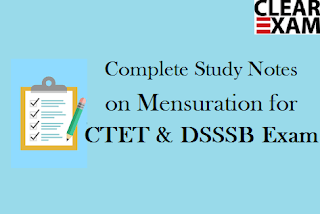## Study notes on Mensuration for CTET and DSSSB Exams

Here we are providing you Study notes on Mensuration which will be helpful for Upcoming CTET and DSSSB Exams.

Solid Figures :- All figures which have three dimensions i.e. length, breadth and height are known as solid figures. These include cuboid, cube and cylinder figures. Under this we study about volume and surface area of these figures.

What is volume :- Space occupied by an object is called as volume' of that perticular object. It is always measured in cube units.

For Example :- If length breadth and height of a box are 8cm, 3cm, and 4 cm, respectively, then volume of the box will be
length x breadth x height = 8×3×4 = 96cm3

Surface Area :-  Surface are of a solid body is the area of all of its surfaces together. Surface area is measured in square unit.

For Example :- A cube has 6 surfaces and each surface is in a square like shape. Therefore, its surface area will be 6a2 sq. unit, where as a2 is the area of each surface of the cube.

Solid figures :

Cube - A solid body possess 6 equal faces with equal length, breadth and height is called a cube. In fact, each face of a cube is a square. A cube has 6 surface, 8 corner and 12 sides.
(a) Volume of the cube = a3
(b) Whole surface of a cube = 6a2
(c) Diagonal of the cube =
where a = side (or edge) of the cube.

EX- Find the volume and surface area of cube whose edge is 6 metre.
Solution:-
Volume of cube (side)3
=(6)3=216m3
Whole surface of a cube = 6×side
=6×6=36m2

Cuboid :- A rectangular solid body having 6 rectangular faces, is called a cuboid.

(i) Volume of the cuboid = lbh
(ii) Whole surface or surface area of the cuboid = 2 (lb+bh+lh)
Where l = length, b=breadth, h=height.
(A) A box with lid has its shape like cuboid, then
(1) External length = internal length + 2 (thickness)
(3) External height = internal height + 2 (thickness)
(B) A box without lid has it shape like cuboid or cube, then
(1) External length = Internal length + 2 (thickness)
(3) External height = Internal height + thickness.

Ex- Find the volume and surface area of a cuboid 9 m long, 7m broad and 4m height.
Solution:
Volume of the cuboid
= 252 m3
Surface area = 2 (lb + bh + lh)
=2(97+74+94)
=2(63+28+36)
=254m2

T    Thanks

Team clear ctet.Want to Know More
Please fill in the details below:

Name

ltr
item
Clear CTET - Coaching Institute for CTET, DSSSB and KVS: Study notes on Mensuration for CTET and DSSSB Exams
Study notes on Mensuration for CTET and DSSSB Exams
https://4.bp.blogspot.com/-Znz4JJKR6q8/WcN8SBLTjKI/AAAAAAAAAQA/ysxh4LHGLTsPZDobMxK5btF3T8yQlLwVACLcBGAs/s320/Study%2BNotes%2Bon%2BMensuration.png
https://4.bp.blogspot.com/-Znz4JJKR6q8/WcN8SBLTjKI/AAAAAAAAAQA/ysxh4LHGLTsPZDobMxK5btF3T8yQlLwVACLcBGAs/s72-c/Study%2BNotes%2Bon%2BMensuration.png
Clear CTET - Coaching Institute for CTET, DSSSB and KVS
https://www.clearctet.com/2017/09/study-notes-on-mensuration-for-ctet-and.html
https://www.clearctet.com/
https://www.clearctet.com/
https://www.clearctet.com/2017/09/study-notes-on-mensuration-for-ctet-and.html
true
5140436504468951946
UTF-8